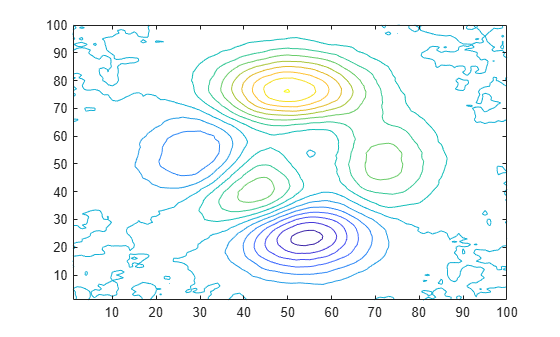Smooth Data with Convolution

You can use convolution to smooth 2-D data that contains high-frequency components.

Create 2-D data using the peaks function, and plot the data at various contour levels.

Z = peaks(100);
levels = -7:1:10;
contour(Z,levels)Inject random noise into the data and plot the noisy contours.

Znoise = Z + rand(100) - 0.5;
contour(Znoise,levels)The conv2 function in MATLAB® convolves 2-D data with a specified kernel whose elements define how to remove or enhance features of the original data. Kernels do not have to be the same size as the input data. Small-sized kernels can be sufficient to smooth data containing only a few frequency components. Larger sized kernels can provide more precision for tuning frequency response, resulting in smoother output.

Define a 3-by-3 kernel K and use conv2 to smooth the noisy data in Znoise. Plot the smoothed contours. The 'same' option in conv2 makes the output the same size as the input.

K = (1/9)*ones(3);
Zsmooth1 = conv2(Znoise,K,'same');
contour(Zsmooth1, levels)Smooth the noisy data with a 5-by-5 kernel, and plot the new contours.

K = (1/25)*ones(5);
Zsmooth2 = conv2(Znoise,K,'same');
contour(Zsmooth2,levels)